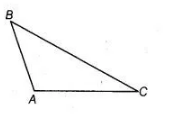# Prove that in a triangle,`
Question:

Prove that in a triangle, other than an equilateral triangle, angle opposite the longest side is greater than 2/3 of a right angle.

Solution:

Consider $\triangle A B C$ in which $B C$ is the longest side.

To prove $\angle A=\frac{2}{3}$ right angle

Proof $\ln \triangle A B C, \quad B C>A B$.

[consider $B C$ is the largest side]$\Rightarrow \quad \angle A>\angle C \quad \ldots(i)$

[angle opposite the longest side is greatest]

and $\quad B C>A C$

$\Rightarrow \quad \angle A>\angle B .$.....(ii)

[angle opposite the longest side is greatest]

On adding Eqs. (i) and (ii), we get

$2 \angle A>\angle B+\angle C$

$\Rightarrow \quad 2 \angle A+\angle A>\angle A+\angle B+\angle C \quad$ [adding $\angle A$ both sides]

$\Rightarrow \quad 3 \angle A>\angle A+\angle B+\angle C$

$\Rightarrow$ $3 \angle A>180^{\circ}$ [sum of all the angles of a triangle is $180^{\circ}$ ]

$\Rightarrow \quad \angle A>\frac{2}{3} \times 90^{\circ}$

i.e., $\angle A>\frac{2}{3}$ of a right angle Hence proved.